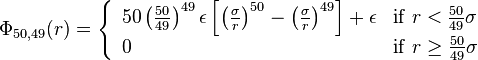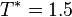# Pseudo hard sphere potential$\Phi_{\rm 50,49} (r) = \left\{ \begin{array}{ll} 50 \left( \frac{50}{49} \right)^{49} \epsilon \left[ \left(\frac{\sigma}{r} \right)^{50}- \left( \frac{\sigma}{r}\right)^{49} \right] + \epsilon & {\rm if} \; r < \frac{50}{49} \sigma \\ 0 & {\rm if} \; r \ge \frac{50}{49} \sigma \end{array} \right.$
Molecular dynamics simulations of this idealised model, when performed at a reduced temperature of$T^*=1.5$ reproduces results for the hard sphere model (for example, the Carnahan-Starling equation of state), which is generally only amenable to Monte Carlo simulations, or advanced techniques such as Event-driven molecular dynamics.